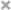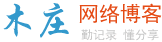# js中常见的一些表单验证正则表达式

`^[0-9]*\$`

`^\d{n}\$`

`^\d{n,}\$`

`^\d{m,n}\$`

`^(0|[1-9][0-9]*)\$`

`^[0-9]+(.[0-9]{2})?\$`

`^[0-9]+(.[0-9]{1,3})?\$`

`^\+?[1-9][0-9]*\$`

`^\-[1-9][0-9]*\$`

`^\d+\$`

`^((-\d+)|(0+))\$`

`^.{3}\$`

`^[A-Za-z]+\$`

`^[A-Z]+\$`

`^[a-z]+\$`

`^[A-Za-z0-9]+\$`

`^\w+\$`

`^[a-zA-Z]\w{5,17}\$`

`[^%&',;=?\$\x22]+`

`^[\u4e00-\u9fa5],{0,}\$`

`^\w+[-+.]\w+)*@\w+([-.]\w+)*\.\w+([-.]\w+)*\$`

`^http://([\w-]+\.)+[\w-]+(/[\w-./?%&=]*)?\$ ；^[a-zA-z]+://(w+(-w+)*)(.(w+(-w+)*))*(?S*)?\$`

`^(\(\d{3,4}\)|\d{3,4}-)?\d{7,8}\$`

`^\d{15}|\d{}18\$`

`^(0?[1-9]|1[0-2])\$`

`^((0?[1-9])|((1|2)[0-9])|30|31)\$`

`^-?\d+\$`

`^\d+(\.\d+)?\$`

`^(([0-9]+\.[0-9]*[1-9][0-9]*)|([0-9]*[1-9][0-9]*\.[0-9]+)|([0-9]*[1-9][0-9]*))\$`

`^((-\d+(\.\d+)?)|(0+(\.0+)?))\$`

`^(-(([0-9]+\.[0-9]*[1-9][0-9]*)|([0-9]*[1-9][0-9]*\.[0-9]+)|([0-9]*[1-9][0-9]*)))\$`

`^(-?\d+)(\.\d+)?\$`

js与css的区别是什么

console.log()的作用

10款好看且实用的文字动画特效，让你的页面更吸引人！

javascript如何定时自动关闭页面

h5+js实现本地文件读取和写入

css和js后加问号和数字有什么用

javascript 和 dart 的区别

js concat什么时候用

three.js使用gpu选取物体并计算交点位置

js怎么换行### 评论

• 欢迎访问木庄网络博客
• 可复制：代码框内的文字。
• 方法：Ctrl+C。